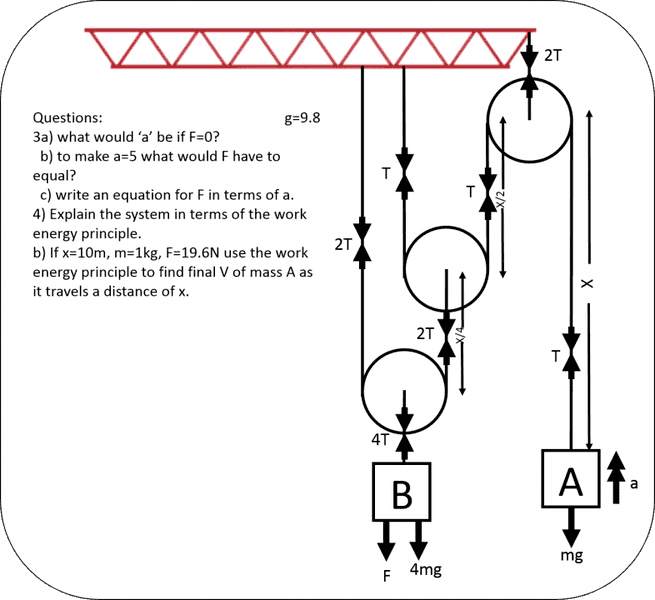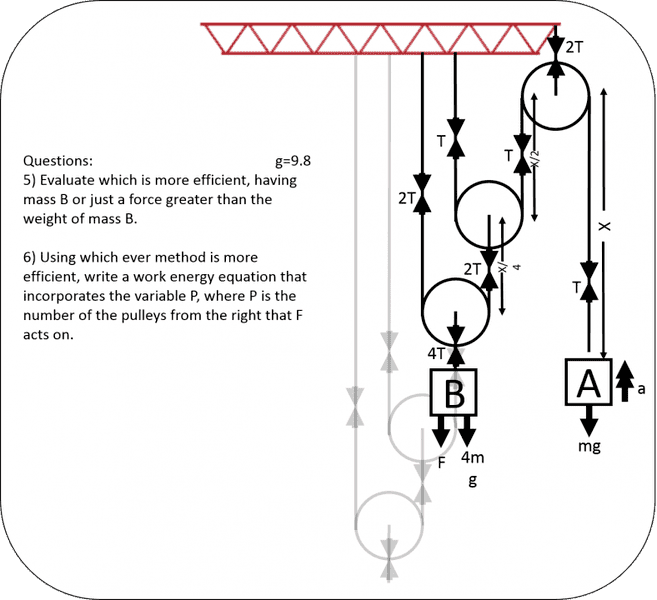# Pulley question -- 2 pulleys and 2 weights

THIS THREAD WAS INCORRECTLY PLACED IN THE CLASSICAL PHYSICS FORUM, SO THERE IS NO TEMPLATE

Hi, everyone.
my teacher stuck me with these questions and i am need of a little help answering question 2.

here's my answers to question 1 first.
1a) a=0, as system would be in equilibrium when F=0
b) F=5mgN, as for a=5m/s the acceleration of B has to equal 2.5m/s and as its in equilibrium F has just got to equal m*a which is 2.5*2mg=5mgN
c) F=mga

i think my answers are right but if im making a simple mistake let me know.

now my attempts at 2.

2a) first, B has to x/2 above the ground for the full system to work so original potential energy is 2mgx/2=mgx assuming A starts on the ground. now its the following kenetic energy bits that are confusing me because there will be two different V's one for A and one for B. VA = 2VB so assuming no energy gets lost to heat via friction whatever all of the gravitational energy plus energy input from F has to turn into kinetic energy so im thinking.

mgx + Fx = 1/2mVA 2 + mVB 2

which kind of works if your given enough information. but part B doesn't i don't think.

2b) substituting values into my answer to 2a

98 + 196 = 1/2[ VA 2 + 2VB 2 ]
so VA 2 + 2VB 2 = 588N
and VA = 2VB
so with further manipulation i get VA = 19.8m/s

but using logic from question 1. if F=19.6 and B has a mass of 2kg, the acceleration of B = 9.8m/s 2 therefore acceleration of A = 19.6m/s 2 and then using SUVAT.

s=10m u=0 v=? a=19.6 m/s 2
v 2 = u 2 +2as
v = 23.2m/s

which is different. iv tried multiple times and i can't figure out what im doing wrong. im pretty sure its the WEP part not the SUVAT part as im better at that but i do not know.

#### Attachments

•mech question.png
43.5 KB · Views: 1,479
Last edited by a moderator:

SteamKing
Staff Emeritus
Homework Helper
It would be nice if you had posted the text of the questions as well.

As your post reads now, we have no idea if you are correct or not.

It would be nice if you had posted the text of the questions as well.

As your post reads now, we have no idea if you are correct or not.

iv updated it now so the picture of the question is on. sorry about that. it quite late haha

haruspex
Homework Helper
Gold Member
2020 Award
Write out the full force/acceleration equations for the two masses, including T as a common unknown.
Taking shortcuts often leads to errors.

Write out the full force/acceleration equations for the two masses, including T as a common unknown.
Taking shortcuts often leads to errors.

okay, here i go.

For mass A: T-mg = 5m =X2=> 2T-2mg = 10m
For mass B: F+2mg-2T= -2.5(2m) = -5m

F=5m

is that correct?

haruspex
Homework Helper
Gold Member
2020 Award
okay, here i go.

For mass A: T-mg = 5m =X2=> 2T-2mg = 10m
For mass B: F+2mg-2T= -2.5(2m) = -5m

F=5m

is that correct?
Check your signs in the mass B equation. Think which way the acceleration is.

Check your signs in the mass B equation. Think which way the acceleration is.

i have taken up to be my positive direction so a is positive for A and negative for B.

is that not right?

haruspex
Homework Helper
Gold Member
2020 Award
i have taken up to be my positive direction so a is positive for A and negative for B.

is that not right?
The left hand side of your mass B equation takes down to be positive.

The left hand side of your mass B equation takes down to be positive.
okay then. would that them make F=15m ?

haruspex
Homework Helper
Gold Member
2020 Award
okay then. would that them make F=15m ?
Yes. But you should include units since the 15 is not dimensionless.

Does that then make the answer to 1c) F=3ma N ?

haruspex
Homework Helper
Gold Member
2020 Award
Does that then make the answer to 1c) F=3ma N ?
Yes, but this time you should leave out the units. That's because m is a mass and a an aceleration in unspecified units, so it's just F=3ma.

so was my WEP question right?

haruspex
Homework Helper
Gold Member
2020 Award
B has to x/2 above the ground for the full system to work
Do you mean it has to start x/2 above the ground? It certainly needs to be at least that.
original potential energy is 2mgx/2=mgx
You can't say what the original PE is unless you define a zero level. The loss in PE by B will be mgx.
mgx + Fx = 1/2mVA 2 + mVB 2
How far does F move?

assume the zero lvl is at the base of mass A and B is exactly X/2 above the ground.
the PE of the entire system is then: 2mgx/2 = mgx
the energy by F is: Fx/2
KE of A afterwards is 1/2*m*va2
KE of B afterwards is 1/2*2m*(vb/2)2 = m*(vb/2)2

as vb = 1/2va

KE of B can also be written as: m*(va/4)2 = mva2/16

so the equation should be:
mgx + Fx/2 = 1/2mva2 + mva2/16

which can be simplified to:
mgx + Fx/2 = 9/16mv2

is that right or am i missing somthing?

haruspex
Homework Helper
Gold Member
2020 Award
KE of B afterwards is 1/2*2m*(vb/2)2
Why vb/2?

i was thinking as it is half Va but then i did it again. does that then make the end, mgx + Fx/2 = 3/4mv2 is that right?

haruspex
Homework Helper
Gold Member
2020 Award
i was thinking as it is half Va but then i did it again. does that then make the end, mgx + Fx/2 = 3/4mv2 is that right?
Yes.

for part 2b im still getting different answers depending on which method i use.

if F=3ma, 19.6=3(1)a, a=6.53.
using v2 = u2+2as
v2 = 2(6.53)(10) = 130.67
so, v= 11.43 m/s

but if we use mgx + Fx/2 = 3/4mv2
1(9.8)(10) + 19.6(10/2) = 3/4(1)v2
196 = 3/4v2
v2=261.33
v = 16.17 m/s

whats wrong?

haruspex
Homework Helper
Gold Member
2020 Award
for part 2b im still getting different answers depending on which method i use.

if F=3ma, 19.6=3(1)a, a=6.53.
using v2 = u2+2as
v2 = 2(6.53)(10) = 130.67
so, v= 11.43 m/s

but if we use mgx + Fx/2 = 3/4mv2
1(9.8)(10) + 19.6(10/2) = 3/4(1)v2
196 = 3/4v2
v2=261.33
v = 16.17 m/s

whats wrong?
I missed another error in the OP.
Think again about the total change in PE.

would mass A also gain PE
so mgx + Fx/2 = 3/4mv2 + mgx
which would leave you with Fx/2 = 3/4mv2

19.6(10)/2 = 3/4(1)v2
98 = 3/4v2
v2 = 130.67
v = 11.43

which is the same as the suvat and forces one. :) we did it

haruspex
Homework Helper
Gold Member
2020 Award
would mass A also gain PE
so mgx + Fx/2 = 3/4mv2 + mgx
which would leave you with Fx/2 = 3/4mv2

19.6(10)/2 = 3/4(1)v2
98 = 3/4v2
v2 = 130.67
v = 11.43

which is the same as the suvat and forces one. :) we did it
Yes.

it was right and i then received more questionswhat iv noticed is that the questions are the same, so this is basically a check before i hand in my answers as hopefully ill get them right.
iv also noticed B will never be as low as A due to the ropes making a infinite series upto 1 or x but this time B can only move x/4 if A is on the ground.

okay question time.

3a) a = 0 as in equilibrium again

b) do c) first and put a = 5 gives F = 25m N

c) F+4mg-4T = 4ma/4 = ma
T-mg = ma ==> 4T-4mg = 4ma
so F = 5ma

4) 4mgx/4 + Fx/4 = 1/2(4m)(v/4)2 + 1/2mv2 + mgx
mgx + Fx/4 = mv2/8 + 1/2 mv2 + mgx
Fx/4 = 5/8 mv2

4b) 19.6(10)/4 = 5/8(1)v2
49 = 5/8v2
v2 = 78.4
v = 8.85 m/s

haruspex
Homework Helper
Gold Member
2020 Award
it was right and i then received more questions
View attachment 86870
what iv noticed is that the questions are the same, so this is basically a check before i hand in my answers as hopefully ill get them right.
iv also noticed B will never be as low as A due to the ropes making a infinite series upto 1 or x but this time B can only move x/4 if A is on the ground.

okay question time.

3a) a = 0 as in equilibrium again

b) do c) first and put a = 5 gives F = 25m N

c) F+4mg-4T = 4ma/4 = ma
T-mg = ma ==> 4T-4mg = 4ma
so F = 5ma

4) 4mgx/4 + Fx/4 = 1/2(4m)(v/4)2 + 1/2mv2 + mgx
mgx + Fx/4 = mv2/8 + 1/2 mv2 + mgx
Fx/4 = 5/8 mv2

4b) 19.6(10)/4 = 5/8(1)v2
49 = 5/8v2
v2 = 78.4
v = 8.85 m/s
Looks right.

he had more. just checking again for 5 and need help on 6.5) we have an equation to find V when B is there.

Fx/4 = 5/8mv2

we can then work out an equation for when B isnt there:

Fx/4 = 1/2mv2 + mgx

now F has to be greater than the weight of B as the question says so let F = 100N, m=1KG, and x = 10m.

with B:
100(10)/4 = 5/8(1)v2
250 = 5/8v2
v2 = 400
v = 20 m/s

without B:
100(10)/4 = 1/2(1)v2 + 1(9.8)(10)
152 = 1/2v2
v2 = 304
v = 17.44 m/s

therefore with B is more efficient.

6) now as its the method with B i want to assume B changes as P increases so that the system remains in equalibrium without F as it did between questions 1-2 and 3-4.
if that's the case i worked out the mass of B = 2p-1
it would also have less to travel, x/2p-1 m i think?

so would it look something like:

2p-1mgx/2p-1 + Fx/2p-1 = 1/2m(v/2p-1)2 + 1/2mv2 + mgx

mgx + Fx/2p-1 = 1/2mv2/22p-2 + 1/2mv2 + mgx

Fx/2p-1 = mv2/22p-1 + 1/2mv2

Fx/2p-1 = (2+22p-1)(mv2)/22p

now that doesn't seem right at all so i would for you to check it over and correct me please haha

thanks.